##Regular Articles

# Fast Algorithm for Monitoring Data Streams by Using Hidden Markov Models

## Abstract

We describe a fast algorithm for exact and efficient monitoring of streaming data sequences. Our algorithm, SPIRAL-Stream, is a fast search method for finding the best model among a set of candidate hidden Markov models (HMMs) for given data streams. It is based on three ideas: (1) it clusters model states to compute approximate likelihoods, (2) it uses several granularities of clustering and approximation level of likelihood values in search processing, and (3) it focuses on the efficient computation of only promising likelihoods by pruning out low-likelihood state sequences. Experiments verified its effective­ness and showed that it was more than 490 times faster than the naive method.

† NTT Cyber Space Laboratories Yokosuka-shi, 239-0847 Japan

## 1. Introduction

Significant applications that use hidden Markov models (HMMs), including traffic monitoring and traffic anomaly detection, have emerged. The goal of this study is efficient monitoring of streaming data sequences by finding the best model in an exact way. Although numerous studies have been published in various research areas, this is, to the best of our knowledge, the first study to address the HMM search problem in a way that guarantees the exactness of the answer.

### 1.1 Problem definition

Increasing the speed of computing HMM likelihoods remains a major goal for the speech recognition community. This is because most of the total processing time (30–70%) in speech recognition is used to compute the likelihoods of continuous density HMMs. Replacing continuous density HMMs by discrete HMMs is a useful approach to reducing the computation cost . Unfortunately, the central processing unit cost still remains excessive, especially for large datasets, since all possible likelihoods are computed.

Recently, the focus of data engineering has shifted toward data stream applications . These applications handle continuous streams of input from external sources such as a sensor. Therefore, we address the following problem in this article:

Problem Given an HMM set and a subsequence of data stream X = (x1, x2, ..., xn), where xn is the most recent value, identify the model whose state sequences have the highest likelihood, estimated with respect to X, among the set of HMMs by monitoring the incoming data stream.

Key examples of this problem are traffic monitoring ,  and anomaly detection , .

### 1.2 New contribution

We have previously proposed a method called SPIRAL that offers fast likelihood searches for static sequences . In this article*, it is extended to data streams; this extended approach, called SPIRAL-Stream, finds the best model for data streams . To reduce the search cost, we (1) reduce the number of states by clustering multiple states into clusters to compute the approximate likelihood, (2) compute the approximate likelihood with several levels of granularity, and (3) prune low-likelihood state sequences that will not yield the best model. SPIRAL-Stream has the following attractive characteristics based on the above ideas:

- High-speed searching: Solutions based on the Viterbi algorithm are prohibitively expensive for large HMM datasets. SPIRAL-Stream uses carefully designed approximations to efficiently identify the most likely model.

- Exactness: SPIRAL-Stream does not sacrifice accuracy; it returns the highest likelihood model without any omissions.

To achieve high performance and find the exact answer, SPIRAL-Stream first prunes many models by using approximate likelihoods at a low computation cost. The exact likelihood computations are performed only if absolutely necessary, which yields a drastic reduction in the total search cost.

The remainder of this article is organized as follows. Section 2 overviews some background of HMMs. Section 3 introduces SPIRAL-Stream and shows how it identifies the best model for data streams. Section 4 presents the results of our experiments. Section 5 is a brief conclusion.

 *1 The present article is basically a shortened version of ref. . Readers who would like more details, should refer to ref. .

## 2. HMMs

In this section, we explain the basic theory of HMMs.

### 2.1 Definitions

Unlike the regular Markov model, in which each state corresponds to an observable event, an HMM is used when there is a set of unobserved, thus hidden, states and the observation is a probabilistic function of the state. Let {ui} (i = 1, ..., m) be a set of states. An HMM is composed of the following probabilities:

- Initial state probability π = {πi}: The probability of the state being ui (i = 1, ..., m) at time t.

- State transition probability a = {aij}: The probability of the state transiting from state ui to uj.

- Symbol probability b(v) = {b(v)}: The probability of symbol v being output from state ui.

HMMs are classified by the structure of the transition probability. Ergodic HMMs, or fully connected HMMs, have the property that every state can be reached from every other state. Another type of HMM is the left-right HMM; its state transitions have the property that, as time increases, the state number increases or stays the same.

### 2.2 Viterbi algorithm

The well-known Viterbi algorithm is a dynamic programming algorithm for HMMs that identifies the most-likely state sequence with the maximum probability and estimates its likelihood given an observed sequence. The state sequence, which gives the likelihood, is called the Viterbi path. For a given model, the likelihood P of X is computed as follows: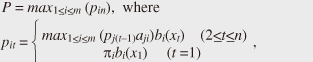where m is the length of the sequence, n is the number of states, and pit is the maximum probability of state ui at time t. The likelihood is computed on the basis of the trellis structure shown in Fig. 1, where states lie on the vertical axis and sequences are aligned along the horizontal axis. The likelihood is computed using the dynamic programming approach that maximizes the probabilities from previous states (i.e., each state probability is computed using all previous state probabilities, associated transition probabilities, and symbol probabilities).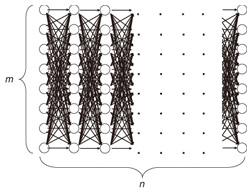Fig. 1. Trellis structure.

The Viterbi algorithm generally needs O(nm2) time since it compares m transitions to obtain the maximum probability for every state; that is, it requires O(nm2) in each time tick. The naive approach to monitoring data streams is to perform this procedure each time a sequence value arrives. However, considering the high frequency with which new values will arrive, more efficient algorithms are needed.

## 3. Finding the best model for data streams: SPIRAL-Stream

In this section, we discuss how to handle data streams.

### 3.1 Ideas behind SPIRAL-Stream

Our solution is based on three ideas: likelihood approximation, multiple granularities, and transition pruning. These are outlined below in this subsection and explained in more detail in subsections 3.2–3.4.

### (1) Likelihood approximation

In a naive approach to finding the best model among a set of candidate HMMs, the Viterbi algorithm would have to be applied to all the models, but the algorithm’s cost would be too high when it is applied to the entire set of HMMs. Therefore, we introduce approximations to reduce the high cost of the Viterbi algorithm solution. Instead of computing the exact likelihood of a model, we approximate the likelihood; thus, low-likelihood models are efficiently pruned.

The first idea is to reduce the model size. For given m states and granularity g, we create m/g states by merging similar states in the model (Fig. 2(a)), which requires O(nm2/g2) time to obtain the approximate likelihoods instead of the O(nm2) time demanded by the Viterbi algorithm solution. We use a clustering approach to find groups of similar states and then create a compact model that covers the groups. We refer to it as the degenerate model.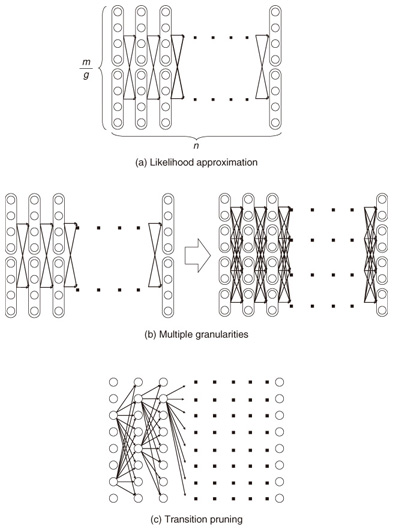Fig. 2. Basic ideas behind SPIRAL.

### (2) Multiple granularities

Instead of creating degenerate models at just one granularity, we use multiple granularities to optimize the tradeoff between accuracy and comparison speed. As the size of a model increases, its accuracy improves (i.e., the upper bounding likelihood decreases), but the likelihood computation time increases. Therefore, we generate models at granularity levels that form a geometric progression: g = 1,2,4,..., m, where g = 1 gives the exact likelihood while g = m means the coarsest approximation. We then start from the coarsest model and gradually increase the size of the models to prune unlikely models; this improves the accuracy of the approximate likelihood as the search progresses (Fig. 2(b)).

### (3) Transition pruning

Although our approximation technique can discard unlikely models, we still rely on exact likelihood computation to guarantee the correctness of the search results. Here, we focus on reducing the cost of this computation.

The Viterbi path shows the state sequence from which the likelihood is computed. Even though the Viterbi algorithm does not compute the complete set of paths, the trellis structure includes an exponential number of paths. Clearly, exhaustive exploration of all paths is not computationally feasible, especially for a large number of states. Therefore, we ask the question: Which paths in the structure are not promising to explore? This can be answered by using a threshold (say θ).

Our search algorithm that identifies the best model maintains the candidate (i.e., best-so-far) likelihood before reporting the final likelihood. Here, we use θ as the best-so-far highest likelihood. θ is updated, i.e., increased, when a more promising model is found during search processing. Note that we assume that no two models have exactly the same likelihoods.

We exclude the unlikely paths in the trellis structure by using θ, since θ never decreases during search processing. If the upper bounding likelihood of paths that pass through a state is less than θ, that state cannot be contained in the Viterbi path, and we can safely discard these paths, as shown in Fig. 2(c).

### 3.2 Likelihood approximation

Our first idea involves clustering states of the original models and computing upper bounding likelihoods to achieve reliable model pruning.

#### 3.2.1 State clustering

We reduce the size of the trellis structure by merging similar states in order to compute likelihoods at low computation cost. To achieve this, we use a clustering approach. Given granularity g, we try to find m/g clusters from among the m original states. First, we describe how to compute the probabilities of a new degenerate model; then, we describe our clustering method.

We merge all the states in a cluster and create a new state. For the new state, we choose the highest probability among the probabilities of the states to compute the upper bounding likelihood (described in subsection 3.2.2). We obtain the probabilities of new state Uc by merging all the states in cluster C as follows.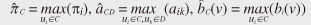We use the following vector of features Fi to cluster state ui.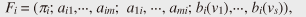where s is the number of symbols. We choose this vector to reduce the approximation error. The highest probabilities are the probabilities of a new state. Therefore, the greater the difference in probabilities possessed by the two states, the greater the difference in the vectors becomes. Thus, a good clustering arrangement can be found by using this vector.

In our experiments, we used the well-known k-means method to cluster states where the Euclidean distance is used as a distance measure. However, we could exploit BIRCH  instead of the k-means method, the L1 distance as a distance measure, or singular value decomposition to reduce the dimensionality of the vector of features. The clustering method is completely independent of SPIRAL-Stream and is beyond the scope of this article.

#### 3.2.2 Upper bounding likelihood

We compute approximate likelihood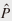from degenerate models that have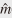(=m/g)states. Given a degenerate model, we compute its approximate likelihood as follows: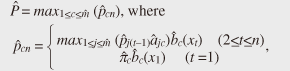whereis the maximum probability of states.

Theorem 1 For any HMM model, P ≤holds.

Proof Omitted owing to space limitations.

Theorem 1 provides SPIRAL-Stream with the property of finding the exact answer .

### 3.3 Multiple granularities

The algorithm presented in subsection 3.2 uses a single level of granularity to compute the approximate likelihood of a degenerate model. However, we can also exploit multiple granularities. Here, we describe the gradual refinement of the likelihood approximation with multiple granularities. In this subsection, we first describe the definition of data streams and then our approach for data streams.

In data stream processing, the time interval of interest is generally called the window and there are three temporal spans for which the values of data streams need to be calculated :

- Landmark window model: In this temporal span, data streams are computed on the basis of the values between a specific time point, called the landmark, and the present.

- Sliding window model: Given sliding window length n and the current time point, the sliding window model computes the subsequence from the prior n − 1 time to the current time.

- Damped window model: In this model, recent data values are more important than earlier ones. That is, in a damped window model, the weights applied to data decrease exponentially into the past.

This article focuses on the sliding window model because it is used most often and is the most general model. We consider a data stream as a time-ordered series of tuples (time point, value). Each stream has a new value available at each time interval, e.g., every second. We assume that the most recent sample is always taken at time n. Hence, a streaming sequence takes the form (..., x1, x2, ..., xn). Likelihoods are computed only with n values from the streaming sequence, so we are only interested in subsequences of the streaming sequence from x1 to xn.

For data streams, we use h + 1 distinct granularities that form a geometric progression gi = 2i (i = 0,1,2, ..., h). Therefore, we generate trellis structures of models that have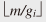states. Here, gh represents the smallest (coarsest) model while g0 corresponds to the original model, which gives the exact likelihood. In the previous our study for static sequences , we first compute the coarsest structure for all models. We then obtain the candidate and the exact likelihood θ. If a model has an approximate likelihood smaller than θ, that model is pruned with no further computation. Otherwise, we compute a finer-grained structure for that model and check whether the approximate likelihood is smaller than θ. We iterate this check until we reach g0.

For data streams, model granularity can be more efficiently decided by referring to the immediately prior granularity for data streams. It is reasonable to expect that the likelihood of the model examined for the subsequence will change little and that we can prune the models efficiently by continuing to use the prior granularities. That is, in the present time tick, the initial granularity is set relative to the finest granularity in the previous time tick at which the model likelihood was computed. If model pruning was conducted at the coarsest granularity, we use this granularity in the next time tick; otherwise, we use the granularity level that is one step down (coarser) as the initial granularity. If the model is not pruned at the initial granularity, the approximate likelihood of a finer-grained structure is computed to check for model pruning against the given θ.

Example If the original HMM has 16 states and the model was pruned with the 1-state model (granularity g4, coarsest), we choose to use the 1-state model (granularity g4) as the initial model in the next time tick; if the model is pruned using the approximate likelihood of 16 states (granularity g0), we select the 8-state model (granularity g1) as the initial model.

### 3.4 Transition pruning

We introduce an algorithm for computing likelihoods efficiently on the basis of the following theorem:

Lemma 1 Likelihoods of a state sequence are monotonically nonincreasing with respect to X in the trellis structure.

Proof Omitted owing to space limitations.

We exploit the above lemma in pruning paths in the trellis structure. We introduce eit, which indicates a conservative estimate of likelihood pit, to prune unlikely paths as follows: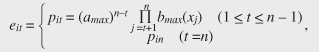where amax and bmax(v) are the maximum values of the state transition probability and symbol probability, respectively:

amax=maxi,j(aij), bmax(v)=maxibi(v),(i=1,...,m; j=1,..., m).

The estimate is exactly the same as the maximum probability of ui when t = n. Estimate eit, the product of the series of the maximum values of the state transition probability and symbol probability, has the upper bounding property assuming that the Viterbi path passes through ui at time t.

Theorem 2 For paths that pass through state ui(i = 1, ..., m) at time t(1 ≤ t ≤ n), pjn ≤ eit holds for any state uj(j = 1, ..., m) at time n.

Proof Omitted owing to space limitations.

This property enables SPIRAL-Stream to search for models exactly.

In search processing, if eit gives a value smaller than θ (i.e., the best-so-far highest likelihood in the search processing for the best model), state ui at time t for the model cannot be contained in the Viterbi path. Accordingly, unlikely paths can be pruned with safety.

### 3.5 Search algorithm

Our approach to data stream processing is shown in Fig. 3. Here, Mi represents the set of models for which we compute the likelihood of granularity gi, and M'i represents the set of models computed with the finest granularity in the previous time tick, gi. SPIRAL-Stream first computes the initial value of θ on the basis of the best model at the last time; it then sets the initial granularity. If a model is pruned at the coarsest granularity at the last time tick, SPIRAL-Stream uses this granularity as the initial granularity. Therefore, we add M'h to Mh in this algorithm. The one-step-lower granularity is used as the initial granularity if the model was not pruned at the coarsest granularity; this procedure is expressed by “add M'i-1 to M'i”.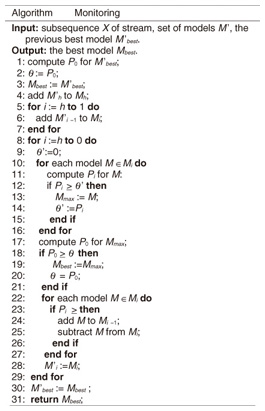Fig. 3. Search algorithm.

### 3.6 Theoretical analysis

In this subsection, we provide a theoretical analysis that shows the accuracy and complexity of SPIRAL-Stream.

Theorem 2 SPIRAL-Stream guarantees the exact answer when identifying the model whose state sequence has the highest likelihood.

Proof Let Mbest be the best model in the dataset and θmax be the exact likelihood of Mbest (i.e., θmax is the highest likelihood). Moreover, let Pi be the likelihood of model M for granularity gi and θ be the best-so-far (highest) likelihood in the search process. From Theorems 1 and 2, we obtain P0 ≤ Pi, for any granularity gi, for any M. For Mbest, θmax ≤ Pi holds. In the search process, since θ is monotonically nondecreasing and θmax ≥ θ, the approximate likelihood of Mbest is never lower than θ, where θ is monotonically nondecreasing. The algorithm discards M if (and only if) θ > Pi. Therefore, the best model Mbest cannot be pruned erroneously during the search process.

## 4. Experimental evaluation

We performed experiments to test SPIRAL-Stream’s effectiveness. We compared SPIRAL-Stream  with the Viterbi algorithm, which we refer to as Viterbi hereinafter, and SPIRAL , which is our previous approach for static sequences.

### 4.1 Experimental data and environment

We used four standard datasets in the experiments.

- EEG: This dataset was taken from a large electroencephalography (EEG) study that examined the EEG correlates of genetic predisposition to alcoholism. In our experiments, we quantized EEG values in 1-µV steps, which resulted in 506 elements.

- Chromosome: We used DNA (deoxyribonucleic acid) strings of human chromosomes 2, 18, 21, and 22. DNA strings are composed of the four letters of the genetic code: A, C, G, and T; however, here we use an additional letter N to denote an unknown letter. Thus, the number of symbols (symbol size) is 5.

- Traffic: This dataset contains loop sensor measurements of the Freeway Performance Measurement System. The symbol size is 91.

- UNIX: We exploited the command histories of 8 UNIX computer users at a university over a two-year period. The symbol size is 2360.

The models were trained by the Baum-Welch algorithm . In our experiments, the sequence length was 256 and possible transitions of a left-right model were restricted to only two states, which is typical in many applications.

We evaluated the search performance mainly by measuring the duration using a wall clock. All experiments were conducted on a Linux quad 3.33 GHz Intel Xeon server with 32 GB of main memory. We implemented our algorithms using the GCC compiler. Each result reported here is the average of 100 trials.

### 4.2 Results of data stream monitoring

We conducted several experiments to test the effectiveness of our approach for monitoring data streams.

#### 4.2.1 Search cost

In Figs. 4(a) and (b), SPIRAL-Stream is compared with Viterbi and with the state-of-the-art approach for data sequences, SPIRAL, which finds the best model for static sequences, in terms of the wall-clock time for various datasets where the number of states and number of models are 100 and 10,000, respectively. As expected, SPIRAL-Stream outperformed the other two algorithms: In particular, SPIRAL-Stream could find the best model up to 490 times faster than the Viterbi algorithm.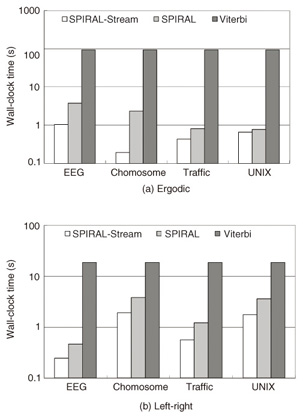Fig. 4. Wall-clock time for monitoring data stream.

#### 4.2.2 Effectiveness of the data stream algorithm

Our stream algorithm (SPIRAL-Stream) automatically changes the granularity and effectively sets the initial candidate to find the best model. To determine the effectiveness of these ideas, we plotted the time at each granularity for SPIRAL-Stream and SPIRAL. The results of time versus granularity in the model search cost for 10,000 models for EEG, where each model has 100 states, are shown in Figs. 5(a) and (b).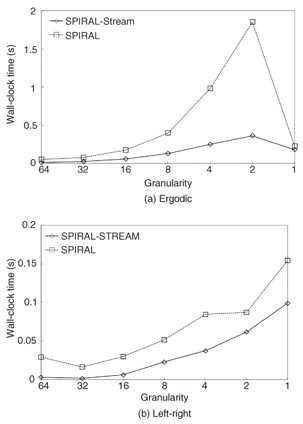Fig. 5. Breakdown of search cost.

SPIRAL-Stream required less computation time at each granularity. Instead of using gh (the coarsest) as the initial granularity for all models, this algorithm sets the initial granularity with the finest granularity at the prior time tick, which ensures that the algorithm reduces the number of models at each granularity. Furthermore, the stream algorithm sets the best model at the prior time tick as the initial candidate, which is expected to remain the answer. As a result, it can find the best model for data streams much more efficiently.

## 5. Conclusion

This article addressed the problem of conducting a likelihood search on a large set of HMMs with the goal of finding the best model for a given query sequence and for data streams. Our algorithm, SPIRAL-Stream, is based on three ideas: (1) it prunes low-likelihood models in the HMM dataset by their approximate likelihoods, which yields promising candidates in an efficient manner; (2) it varies the approximation granularity for each model to maintain a balance between computation time and approximation quality; and (3) it focuses on the efficient computation of only promising likelihoods by pruning out low-likelihood state sequences. Our experiments confirmed that SPIRAL-STREAM worked as expected and quickly found high-likelihood HMMs. Specifically, it was significantly faster (more than 490 times) than the naive implementation.

## References

 S. Sagayama, K. M. Knill, and S. Takahashi, “On the Use of Scalar Quantization for Fast HMM Computation,” Proc. of ICASSP, Vol. 1, pp. 213–216, Detroit, MI, USA, 1995. D. J. Abadi, D. Carney, U. Cetintemel, M. Cherniack, C. Convey, S. Lee, M. Stonebraker, N. Tatbul, and S. B. Zdonik, “Aurora: A New Model and Architecture for Data Stream Management,” Journal of VLDB, Vol. 12, No. 2, pp. 120–139, 2003. P. Bickel, C. Chen, J. Kwon, J. Rice, P. Varaiya, J. R. Pravin, and E. V. Zwet, “Traffic Flow on a Freeway Network,” In Workshop on Nonlinear Estimation and Classification, 2001. J. Kwon and K. Murphy, “Modeling Freeway Traffic with Coupled HMMs,” Tech. Rep., University of California at Berkeley, 2000. T. Lane, “Hidden Markov Models for Human/Computer Interface Modeling,” Proc. of the IJCAI-99 Workshop on Learning About Users, pp. 35–44, 1999. C. Warrender, S. Forrest, and B. A. Pearlmutter, “Detecting Intrusions Using System Calls: Alternative Data Models,” Proc. of the 1999 IEEE Symposium on Security and Privacy, pp. 133–145, Oakland, CA, USA. Y. Fujiwara, Y. Sakurai, and M. Yamamuro, “SPIRAL: Efficient and Exact Model Identification for Hidden Markov Models,” Proc. of KDD’08, pp. 247–255, Las Vegas, NV, USA, 2008. Y. Fujiwara, Y. Sakurai, and M. Kitsuregawa, “Fast Likelihood Search for Hidden Markov Models,” ACM Trans. on Knowledge Discovery from Data (TKDD), Vol. 3, No. 4, 2009. T. Zhang, R. Ramakrishnan, and M. Livny, “BIRCH: An Efficient Data Clustering Method for Very Large Databases,” Proc. of SIGMOD Conference, pp. 103–114, Montreal, Quebec, Canada, 1996. V. Ganti, J. Gehrke, and R. Ramakrishnan, “DEMON: Mining and Monitoring Evolving Data,” IEEE Trans. Knowl. Data Eng., Vol. 13, No. 1, pp. 50–63, 2001. S. E. Levinson, L. R. Rabiner, and M. M. Sondhi, “An Introduction to the Application of the Theory of Probabilistic Functions of a Markov Process to Automatic Speech Recognition,” Bell Syst. Tech. J, Vol. 62, No. 4, pp. 1035–1074, 1982.Yasuhiro Fujiwara Researcher, NTT Cyber Space Laboratories. He received the B.E. and M.E. degrees from Waseda University, Tokyo, in 2001 and 2003, respectively, and the Ph.D. degree from the University of Tokyo in 2012. He joined NTT Cyber Solutions Laboratories in 2003. His research interests include data mining, databases, natural language processing, and artificial intelligence. He received two KDD best paper awards in 2008, IPSJ Best Paper Awards in 2008, IEICE Best Paper Award in 2008, and DASFAA Best Paper Award in 2012. He is a member of the Institute of Physical Society of Japan (IPSJ), Institute of Electronics, Information and Communication Engineers (IEICE), and Database Society of Japan (DBSJ). Yasushi Sakurai Senior Research Scientist, NTT Communication Science Laboratories. He received the B.E. degree from Doshisha University, Kyoto, in 1991 and the M.E. and Ph.D. degrees from Nara Institute of Science and Technology in 1996 and 1999, respectively. He joined NTT Cyber Space Laboratories in 1998. He was a visiting researcher at Carnegie Mellon University, Pittsburgh, PA, USA, during 2004–2005. Since 2007, he has been a senior researcher at NTT Communication Science Laboratories. He received the IPSJ Nagao Special Researcher Award (2007), DBSJ Kambayashi Incentive Award (Young Scientist Award, 2007), and twelve best paper awards, including two KDD best research paper awards (2008 and 2010), IPSJ best paper awards (2004 and 2008), and IEICE Best Paper Award (2008). His research interests include indexing, data mining, and data stream processing.

↑ TOP